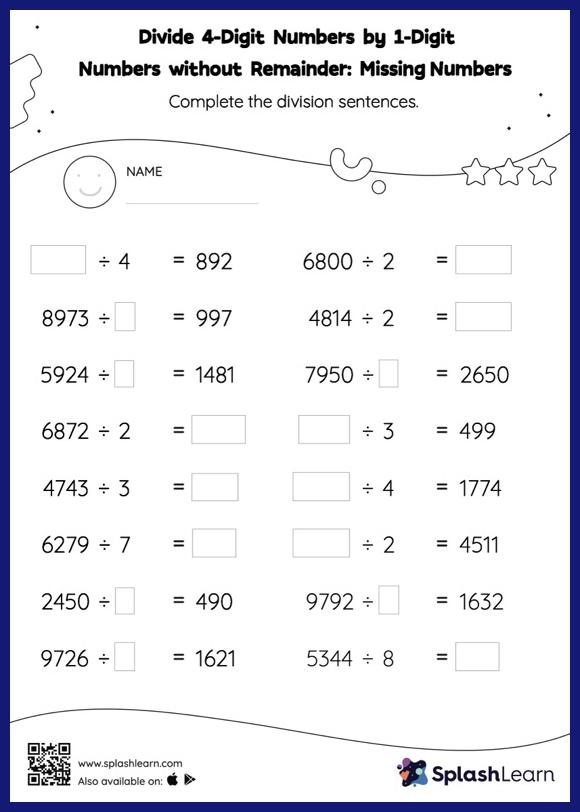# Divide 4-Digit Numbers by 1-Digit Numbers without Remainder: Missing Numbers Worksheet

Home > Divide 4-Digit Numbers by 1-Digit Numbers without Remainder: Missing NumbersThis worksheet challenges young mathematicians to hone their math skills by solving a set of problems on dividing 4-digit numbers by 1-digit numbers without remainder. Students use their prior knowledge of division facts and the long division method to find the missing number in divide 4-digit numbers by 1-digit numbers without remainder worksheet. This set of problems does not have remainders. In each problem, the numbers are laid out in the horizontal format. Students should try to use different strategies involving composing and decomposing numbers to solve these problems. This will help them develop flexibility and fluency.